Home > General Resources > Classroom Activities

# Classroom Activities

The Geometer's Sketchpad is used in classrooms at all levels of the mathematics curriculum. This page gathers information about field-tested classroom activities (including teacher notes, student worksheets, and/or Sketchpad documents), all ready for immediate classroom use. Some of the activities are available in print, some are available free for download, and some are available through third-party links.

## Activity Books

McGraw-Hill Education publishes several curriculum modules that help teachers easily supplement course material with Sketchpad activities, or even use the book as the basis for guided learning. Each module is accompanied by an access code that allows you to download a collection of Sketchpad sketches. Many activities include sketches designed for use with a projector or interactive whiteboard, either for a teacher presentation or a whole-class activity, and Presenter Notes that help you lead a class discussion.

### Exploring Number and Operations in Grades 3–5 with The Geometer's Sketchpad

This collection of Sketchpad activities is an excellent introduction to Sketchpad for both students and teachers, and gives students the opportunity to explore concepts in the Common Core State Standards (grades 3-5) from the domains of operations and algebraic thinking, number and operations in base ten, and number and operations—fractions.

Chapters include: Place Value, Operations, Multiples and Factors, Fractions, Properties of Operations, and Integers and Coordinates.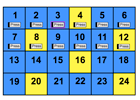### Exploring Geometry and Measurement in Grades 3-5 with The Geometer's Sketchpad

This collection of Sketchpad activities addresses geometry and geometric measurement concepts, and allows for deep engagement with areas designated as critical for Grades 3 and 4 in the Common Core State Standards for Mathematics, including two-dimensional shapes and the structure of area and rectangular array.

Chapters include: Circles, Lines and Angles, Triangles, Quadrilaterals, Transformations, and Area and Perimeter.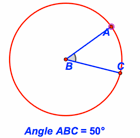### Exploring Ratio, Proportion, and Probability in Grades 6-8 with The Geometer's Sketchpad

This collection of Sketchpad activities allows students to explore concepts and develop skills related to: scatter plots, function graphs, tables, and equations; rate and slope; scale models; and areas of similar shapes.

Chapters include: Rate and Slope, Scale Drawings, Central Tendency, Representing Data, and Probability.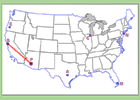### Exploring Expressions and Equations in Grades 6-8 with The Geometer's Sketchpad

This collection of Sketchpad activities gives students the opportunity to explore content from the domain of expressions and equations, as well as functions in Grade 8.

Chapters include: Expressions, Operations, Functions, Graphs of Linear Functions, Nonlinear Functions, Equations and Inequalities, and Polynomials.### Exploring Plane and Solid Geometry in Grades 6-8 with The Geometer's Sketchpad

This collection of Sketchpad activities allows students to explore content from the domain of geometry and develop skills related to: isosceles and right triangles; the Pythagorean Theorem; translations, rotations, and reflections; and more.

Chapters include: Angle Relationships, Triangle Properties, Polygon Properties, Area Formulas, Transformations, and Three-Dimensional Solids and Volume.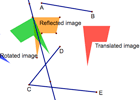### Exploring Algebra 1 with The Geometer's Sketchpad

Activities build upon the strengths of dynamic mathematical representations to enrich the study of first-year algebra, covering such topics as: ratios and exponents; algebraic expressions; and solving equations and inequalities.

Chapters include: Fundamental Operations, Ratios and Exponents, Algebraic Expressions, Solving Equations and Inequalities, Coordinates, Slope, and Distance, Variations and Linear Equations, and Quadratic Equations.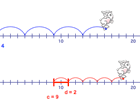### Exploring Geometry with The Geometer's Sketchpad

Dynamic Geometry® software enriches the study of high school geometry, and its use is recommended in the Common Core State Standards. This collection of Sketchpad activities is aligned to the Common Core State Standards, and a full correlation of the activities to the High School Geometry Standards is available for download here. This curriculum module covers virtually every concept studied in high school geometry, including: constructing geometric figures; transformations and tessellations; and modeling with mathematics.

Chapters include: Lines and Angles, Transformations, Symmetry, and Tessellations, Triangles, Quadrilaterals, Polygons, Circles, Area, The Pythagorean Theorem, Similarity, and Trigonometry and Fractals.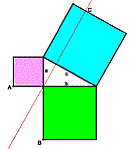### Exploring Algebra 2 with The Geometer's Sketchpad

Demonstrations and activities help users explore Algebra 2 topics, including functions and relations, transformations of functions, systems of equations, matrices, and fitting functions to data.

Chapters include: Functions, Functions and Relations, Systems, Quadratic Functions, Algebraic Transformations, Other Functions, Trigonometric Functions, Probability and Data, and Vectors and Matrices.### Exploring Precalculus with The Geometer's Sketchpad

Activities give users the opportunity to directly experience, through dynamic visualization and manipulation, the topics covered in precalculus, including: polynomial, exponential, and trigonometric functions; parametric functions; polar coordinates; and complex numbers. Also includes activities that are appropriate for use in the first few weeks of a calculus course.

Chapters include: Function Transformations, Circular Functions, Trigonometric Properties, Other Functions, Data and Probability, Vectors and Matrices, Polar Coordinates and Complex Numbers, Sequences and Series, and Introduction to Calculus.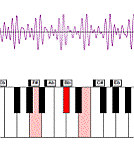### Exploring Calculus with The Geometer's Sketchpad

This collection of Sketchpad activities deepens students' understanding of calculus and covers the fundamental concepts in any first-year calculus course, whether general, Advanced Placement®, or college.

Chapters include: Exploring Change, Exploring Limits, Exploring Derivatives, Exploring Antiderivatives, and Exploring Integrals.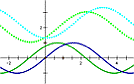## Classroom Activities and Resources

These classroom-ready activities are available here for download, and you may freely duplicate them for use in your own classes.

### The First Week of Calculus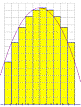Five activities suitable for introducing the main topics of a calculus course. Includes activity handouts and activity sketches for: Instantaneous Rate, Definite Integrals, Trapezoidal Accumulation, Limits with Tables, and Limits with Delta and Epsilon.

### Activities for Young LearnersActivities designed specially for use in elementary and middle school classrooms.  Includes teacher notes and activity sketches for such topics as counting by grouping, measuring, balancing equations, similarity, adding integers, areas of triangles, and circle vocabulary.